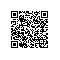# JavaScript函数式编程之深入理解纯函数

var str = 'I am hero';
console.log(str.toUpperCase());	// "I AM HERO"
console.log(str);	//"I am hero"

var str = 'I am hero';
str = str.toUpperCase();	// "I AM HERO"

### 如何理解“相同的输入，永远会得到相同的输出”

var a = 5;
function A(b) {
return a + b;
}
A(5);

A函数是一个纯函数吗？显然非常不纯，在程序执行的过程中，变量a很容易被修改，这就会导致每次调用A(5)的返回值改变。

const a = 5;
function A(b) {
return a + b;
}
A(5);

const obj = {id: 5};
function A(_obj) {
return _obj.id;
}
A(obj);

var obj = {
get id() {
return Math.random();
}
}

const obj = {a: 5};
function A(b) {
return obj.a + b;
}
A(5);

const obj = Object.freeze({a: 5});
function A(b) {
return obj.a + b;
}
A(5);

const obj = Object.freeze({a: {a: 5}});
obj.a.a = 10;
function A(b) {
return obj.a.a + b;
}
console.log(A(5));	// 15，不纯

function foo(x) {
return bar(x);
}

function bar(y) {
return y + 1;
}

foo(1);

function A(a) {
return function(b) {
return a + b;
}
}
var B = A(5);

function A(a) {
a = 0;
return function(b) {
return a + b;
}
}
var B = A(5);

function A(a) {
return function(b) {
return a = a + b;
}
}
var B = A(5);使用钉钉扫一扫加入圈子
+ 订阅